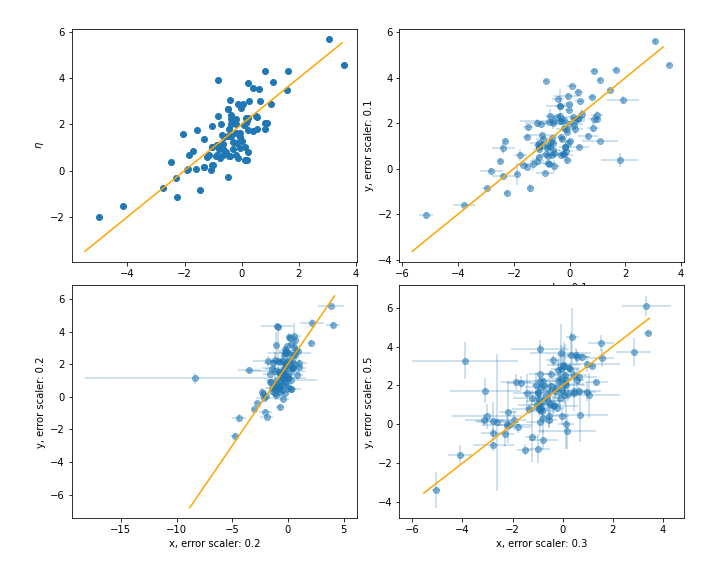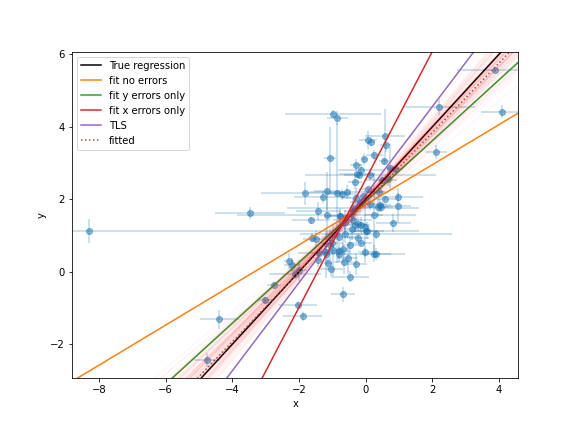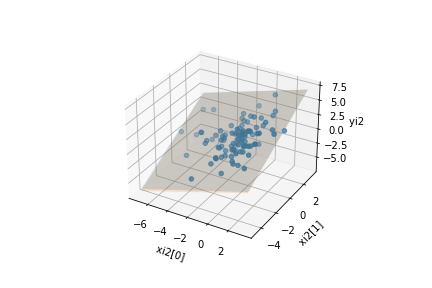# Measurement errors in both dependent and independent variables#

Regression defined as the relation between a dependent variable, $$\eta_i$$, and a set of independent variables, $$\xi_i$$, that describes the expectation value of $$\eta_i$$ given $$\xi_i$$. There may be intrinsic scatter, $$\epsilon_i$$, too.

In most cases, however, what we observe are values $$x_i$$ and $$y_i$$ and associated measurement errors, $$\epsilon_{x,i}$$ and $$\epsilon_{y,i}$$. Following Kelly 2007, we can write the regression relationship as:

$\eta_{i} = \alpha + \beta \xi_{i} + \epsilon_{i}$

and

$x_{i} = \xi_{i} + \epsilon_{x,i}$
$y_{i} = \eta_{i} + \epsilon_{y,i}$

## Data sets used in the examples below#

Use simulation data from Kelly 2007. This simulator, called simulation_kelly is available from astroML.datasets.

The function returns the $$\xi_i$$, $$\eta_i$$, $$x_i$$, $$y_i$$, $$\epsilon_{x,i}$$, $$\epsilon_{y,i}$$ and the input regression coefficients $$\alpha$$ and $$\beta$$ and intrinsic scatter $$\epsilon$$. A total of size values generated, measurement errors are scaled by parameters scalex and scaley following secion 7.1 in Kelly 2007.

from astroML.datasets import simulation_kelly

ksi, eta, xi, yi, xi_error, yi_error, alpha_in, beta_in = simulation_kelly(size=100, scalex=0.2, scaley=0.2,
alpha=2, beta=1, epsilon=(0, 0.75))


### Plot the generated data#

Plot both the simulated $$\xi$$, $$\eta$$ dataset as well the generated observed values of $$x_i$$ and $$y_i$$ including their measurement errors for different values of the scale parameters scalex and scaley.

%matplotlib notebook

import numpy as np
from matplotlib import pyplot as plt
import matplotlib

ksi_0 = np.arange(np.min(ksi) - 0.5, np.max(ksi) + 0.5)
eta_0 = alpha_in + ksi_0 * beta_in

figure = plt.figure(figsize=(10, 8))
bottom=0.1, top=0.95,
hspace=0.1, wspace=0.15)
ax.scatter(ksi, eta)
ax.set_xlabel(r'$\xi$')
ax.set_ylabel(r'$\eta$')

ax.plot(ksi_0, eta_0, color='orange')

for scalex, scaley, axn in [(0.1, 0.1, 222), (0.3, 0.5, 224), (0.2, 0.2, 223)]:
_, _, xi, yi, xi_error, yi_error, _, _ = simulation_kelly(size=100, scalex=scalex, scaley=scaley,
alpha=alpha_in, beta=beta_in, ksi=ksi, eta=eta)

ax.scatter(xi, yi, alpha=0.5)
ax.errorbar(xi, yi, xerr=xi_error, yerr=yi_error, alpha=0.3, ls='')
ax.set_xlabel(f'x, error scaler: {scalex}')
ax.set_ylabel(f'y, error scaler: {scaley}')

x0 = np.arange(np.min(xi) - 0.5, np.max(xi) + 0.5)
y0 = alpha_in + x0 * beta_in
ax.plot(x0, y0, color='orange')### Linear regression with uncertainties in both dependent and independent axes#

The class LinearRegressionwithErrors can be used to take into account measurement errors in both the dependent and independent variables. The implementation relies on the PyMC3 and Theano packages.

Note: The first initialization of the fitter is expected to take a couple of minutes, as Theano performs some code compilation for the underlying model. Sampling for consecutive runs is expected to start up significantly faster.

from astroML.linear_model import LinearRegressionwithErrors

linreg_xy_err = LinearRegressionwithErrors()
linreg_xy_err.fit(xi, yi, yi_error, xi_error)

Auto-assigning NUTS sampler...
Multiprocess sampling (4 chains in 4 jobs)
NUTS: [eta, ksi, mu, tau, int_std, inter, slope]

100.00% [8000/8000 00:16<00:00 Sampling 4 chains, 0 divergences]
Sampling 4 chains for 1_000 tune and 1_000 draw iterations (4_000 + 4_000 draws total) took 24 seconds.

LinearRegressionwithErrors()


## Plot the results#

from astroML.plotting import plot_regressions, plot_regression_from_trace

plot_regressions(ksi, eta, xi, yi, xi_error, yi_error, add_regression_lines=True, alpha_in=alpha_in, beta_in=beta_in)
plot_regression_from_trace(linreg_xy_err, (xi, yi, xi_error, yi_error), ax=plt.gca(), chains=50)Optimization terminated successfully.
Current function value: 113.107250
Iterations: 26
Function evaluations: 52
beta: 0.9615136671425482 alpha: 1.9145807548676619


## Multivariate regression#

For multivariate data (where we fit a hyperplane rather than a straight line) we simply extend the description of the regression function to multiple dimensions. The formalism used in the previous example becomes:

$\eta_i = \alpha + \beta^T \xi_i + \epsilon_i$

where both $$\beta^T$$ and $$\xi_i$$ are now N-element vectors.

### Generate a dataset:#

We use the same function as above to generate 100 datapoints in 2 dimensions. Note that the size of the beta parameter needs to match the dimensions.

ksi2, eta2, xi2, yi2, xi_error2, yi_error2, alpha_in2, beta_in2 = simulation_kelly(size=100, scalex=0.2, scaley=0.2,
alpha=2, beta=[0.5, 1],
multidim=2)


The previously used LinearRegressionwithErrors class can be used with multidimensional data, thus the fitting is done the exact same way as before:

linreg_xy_err2 = LinearRegressionwithErrors()
linreg_xy_err2.fit(xi2, yi2, yi_error2, xi_error2)

Auto-assigning NUTS sampler...
Multiprocess sampling (4 chains in 4 jobs)
NUTS: [eta, ksi, mu, tau, int_std, inter, slope]

100.00% [8000/8000 00:10<00:00 Sampling 4 chains, 0 divergences]
Sampling 4 chains for 1_000 tune and 1_000 draw iterations (4_000 + 4_000 draws total) took 17 seconds.

LinearRegressionwithErrors()


There are several ways to explore the fits, in the following we show a few ways to plot this dataset. As in this example the fitted hyperplane was 2D, we can use a 3D plot to show both the fit and the underlying regession we used to generate the data from. In this 3D plot, the blue plane is the true regression, while the red plane is our fit, that takes into account the errors on the data points.

Other plotting libraries can also be used to e.g. create pairplots of the parameters (e.g. Arviz’ plot_pair function, or Seaborn’s jointplot).

x0 = np.linspace(np.min(xi2)-0.5, np.max(xi2)+0.5, 50)
x1 = np.linspace(np.min(xi2)-0.5, np.max(xi2)+0.5, 50)
x0, x1 = np.meshgrid(x0, x1)

y0 = alpha_in + x0 * beta_in2 + x1 * beta_in2

y_fitted = linreg_xy_err2.coef_ + x0 * linreg_xy_err2.coef_ + x1 * linreg_xy_err2.coef_

import matplotlib.pyplot as plt
from mpl_toolkits.mplot3d import Axes3D
fig = plt.figure()
ax.set_xlabel('xi2 ')
ax.set_ylabel('xi2 ')
ax.set_zlabel('yi2 ')
ax.scatter(xi2, xi2, yi2, s=20)
ax.plot_surface(x0, x1, y0, alpha=0.2, facecolor='blue', label='True regression')
ax.plot_surface(x0, x1, y_fitted, alpha=0.2, facecolor='red', label='Fitted regression')<mpl_toolkits.mplot3d.art3d.Poly3DCollection at 0x1485ac5e0>


### Sampler statistics and traceplots#

The PyMC3 trace is available in the .trace attribute of the class instances (e.g. linreg_xy_err2.trace in the previous example), after we performed the fit. This can be then used for checking for convergence, and generating statistics for the samples. We would refer to use the tools provided by PyMC3, e.g. the traceplot() that takes the trace object as its input. Note that in the multidimensional case, there will be multiple ksi and slope traces in aggreement with the dimensionality of the input xi data, in the traceplot they are plotted with different colours.

import pymc3 as pm
matplotlib.rc('text', usetex=False)

pm.traceplot(linreg_xy_err2.trace)

/Users/bsipocz/.pyenv/versions/3.9.1/lib/python3.9/site-packages/arviz/data/io_pymc3.py:87: FutureWarning: Using from_pymc3 without the model will be deprecated in a future release. Not using the model will return less accurate and less useful results. Make sure you use the model argument or call from_pymc3 within a model context.
warnings.warn(